# Sampling Theorem

### Sampling Theorem

sampling of the signals is the fundamental operation in signal-processing.

A continuous time signal is first converted to discrete-time signal by sampling process.

The sufficient number of samples of the signal must be taken so that the original signal is represented in its samples completely. Also, it should be possible to recover or reconstruct the original signal completely from its samples.

The number of samples to be taken depends on maximum signal frequency present in the signal.

Sampling theorem gives the complete idea about the sampling of signals.

Different types of samples are also taken like ideal samples, natural samples and flat-top samples.

Let us discuss the sampling theorem first and then we shall discuss different types of sampling processes.

The statement of sampling theorem can be given in two parts as:

(i) A band-limited signal of finite energy, which has no frequency-component higher than fm Hz, is completely described by its sample values at uniform intervals less than or equal to 1/ 2fm second apart.

(ii) A band-limited signal of finite energy, which has no frequency components higher than fm Hz, may be completely recovered from the knowledge of its samples taken at the rate of  2fm samples per second.

The first part represents the representation of the signal in its samples and minimum sampling rate required to represent a continuous-time signal into its samples.

The second part of the theorem represents reconstruction of the original signal from its samples. It gives sampling rate required for satisfactory reconstruction of signal from its samples.

Combining the two parts, the sampling theorem may be stated as under:

‘‘A continuous-time signal may be completely represented in its samples and recovered back if the sampling frequency is fs≥ 2fm. Here fs is the sampling frequency and fm is the maximum frequency present in the signal’’.

### Proof of Sampling Theorem

To prove the sampling theorem, we need to show that a signal whose spectrum is band-limited to fm Hz, can be reconstructed exactly without any error from its samples taken uniformly at a rate fs > 2 fHz.

Let us consider a continuous time signal x(t) whose spectrum is band-limited to fHz. This means that the signal x(t) has no frequency components beyond fHz.

Therefore, X(ω) is zero for |ω| > ωm, i.e.,Where ω= 2πfm

Fig.1 (a) shows this continuous-time signal x(t).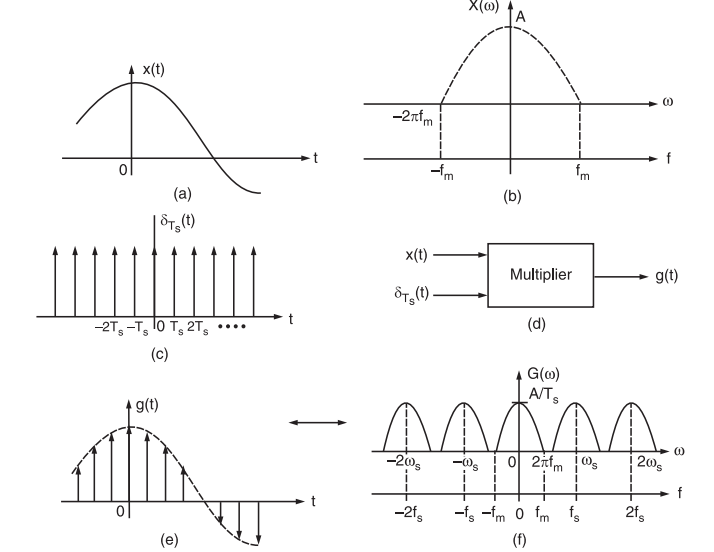Fig.1 (a) A continuous-time signal, (b) Spectrum of continuous-time signal, (c) Impulse train as sampling function, (d) Multiplier, (e) Sampled signal, (f) Spectrum of sampled signal.

Let X(ω) represents its Fourier transform or frequency spectrum as shown in fig.1(b).

Sampling of x(t) at a rate of fHz (i.e.,fs samples per second) can be achieved by multiplying x(t) by an impulse train δTs(t). The impulse train δTs(t) consists of unit impulses repeating periodically every Ts seconds, where Ts = 1/fs.

Fig.1(c) shows this impulse train. This multiplication results in the sampled signal g(t) shown in fig.1(d).

This sampled signal consists of impulses spaced every Ts seconds (the sampling interval). The resulting or sampled signal may be written as under: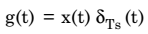…………….. (1)

Again, since the impulse train δTs(t) is a periodic signal of period Ts, it may be expressed as a Fourier series. The Fourier series expansion of impulse-train δTs(t) may be expressed as under:……………. (2)

Here,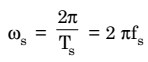Putting the values of δTs(t) from equation (2) in equation (1), the sampled signal can be written as,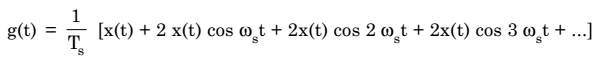…………….. (3)

Now, to obtain G(ω), the Fourier transformation of g(t), we will have to take the Fourier transform of right hand side.

Thus, Fourier transform of x(t) is X(w).

Fourier transform of 2x(t) cos ωst is [X(ω – ωs) + X(ω + ωs)].

Fourier transform of 2x(t) cos 2ωst is [X(ω – 2ωs) + X (ω + 2ωs)] and so on.

Therefore, on taking Fourier transformation, the equation (3) becomes,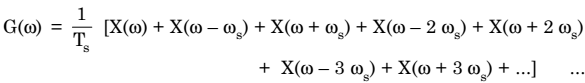.

……………………. (4)

………….. (5)

From equations (4) and (5), it is quite obvious that the spectrum G(ω) consists of X(ω) repeating periodically with period ωs = 2π / Ts rad/sec. or fs = 1 / Ts Hz as shown in fig.1 (f).

Now, if have to reconstruct x(t) from g(t), we must be able to recover X(ω) from G(ω). This is possible if there is no overlap between successive cycles of G(ω). Fig.1 (f) shows that this requires,But, the sampling interval Ts = 1 / fs

Hence,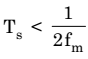Therefore, as long as the sampling frequency fs is greater than twice the maximum signal frequency fm (signal bandwidth, fm), G(ω) will consist of non-overlapping repetitions of X(ω). If this is true, fig.1 (f) shows that x(t) can be recovered from its samples g(t) by passing the sampled signal g(t) through an ideal low-pass filter (LPF) of bandwidth fm Hz. This proves the sampling theorem.